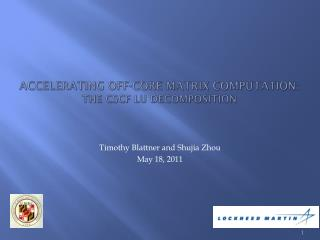# Accelerating off-core matrix computation: the CSCF LU Decomposition - PowerPoint PPT PresentationDownload PresentationAccelerating off-core matrix computation: the CSCF LU Decomposition

Accelerating off-core matrix computation: the CSCF LU Decomposition
Download Presentation## Accelerating off-core matrix computation: the CSCF LU Decomposition

- - - - - - - - - - - - - - - - - - - - - - - - - - - E N D - - - - - - - - - - - - - - - - - - - - - - - - - - -
##### Presentation Transcript

1. Accelerating off-core matrix computation:the CSCF LU Decomposition Timothy Blattner and Shujia Zhou May 18, 2011

2. Acknowledgement This project is sponsored by Lockheed MartinWe would like to thank Joseph Swartz, Sara Hritz, Michael Bellor, Sarah Sellman and Kang Edward for sharing their insight on the CSCF code and Milt Halem for his guidance

3. Outline • Goal • Introduction • Design • Algorithm • Current Implementation • Results • Future Direction

4. Goal • Determine if the use of GPGPU based co-processors provides enough computational acceleration to reduce overall time-to-solution. • Develop generic off-load acceleration model

5. Introduction • Lower-upper Decomposition • Used to solve a system of linear equations • Solves Ax = LUx = b • Slab-based solution • Algorithm uses a forward elimination moving through slabs from left to right • Followed by a backwards substitution going through the slabs from right to left • This is done using a triple buffer solution, • Buffer one is the right hand side slab • Buffer two is the slab being read in • Buffer three is the slab for the current computation • Each element is double precision complex

6. Introduction • Computation solved using a series of FORTRAN routines • Routines - Update_slab and Factor_slab use: • BLAS • ZGEMM • ZTRSM • ZGEMM and ZTRSM accelerated on GPU

7. Technical Challenge • Size of GPU buffer is less than size of one of the CPU buffers • Example: • 1 million unknowns • Contains 1 million rows and 1,250 columns per slab • Total of 800 slabs • Each slab is ~18 GB in size • Largest GPU memory available is 6 GB (Tesla C2070) • Matrices are oblong • Number of rows is much larger than the number of columns

8. Current Design • Solve ZTRSM and ZGEMM on the GPU • CUBLAS • CUDA optimized version of BLAS routines • Domain Decomposition of ZGEMM into GPU buffers • A*B = C A and C  A_GPU Buffer A_CPU Buffer Size = size of A_GPU Buffer COPY COPY N

9. Algorithm • Four Phases: • Phase 1: Baseline Benchmark • Phase 2: Decompose GEMM • Phase 3: Square Matrix Decomposition • Demonstrates effectiveness of GPU on square matrices, and potentially utilizes Fermi’s concurrent kernel execution • Phase 4: Asynchronous Memory Copy • Provides possibility of overlapping PCI express requests, potentially reducing the impact of the bottleneck

10. Current Test Platform • NVIDIA GTX 460 • 336 cores • 1 GB GDDR5 • Intel Q6600 • 4 cores • 2.4 Ghz • 4 GB DDR2 – 800 • 1 TB 7200 RPM Disk

11. Current Implementation • Baseline Benchmark and phase 2 complete for 10,000 unknowns • Implementation in Fortran • Use Fortran to C wrappers provided by NVIDIA for CUBLAS

12. Results (Speedup) Speedup Analysis (CPU time vs GPU time)

13. Results (PCI Express Time) Slabs = Number of Rows / Number of Columns

14. Results (PCI Express Impact)

15. Results (Wall Time)

16. Future Direction • Execute Phase 1 and 2 for up to 1 million unknowns • Run on Tesla C2070 on UMBC Bluegrit cluster • Implement Phase 3 and benchmark • Implement Phase 4 and benchmark • Investigate Factor_slab routine for speedup